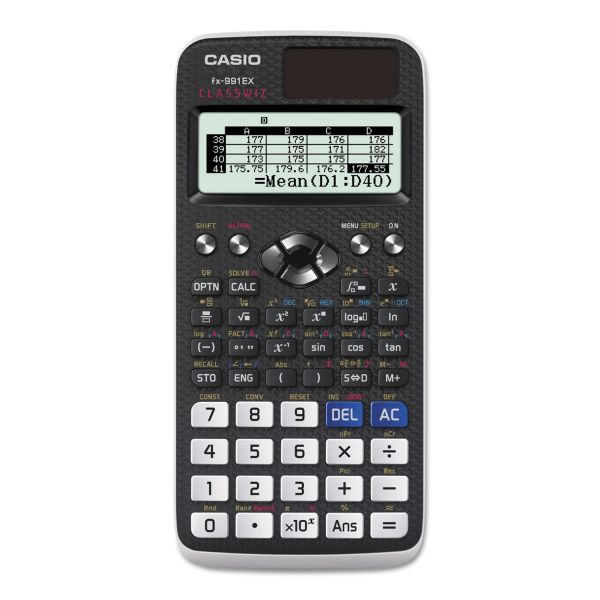# Casio FX-991EX Advanced Scientific Calculator, 15-Digit LCD

Product Number: CSOFX991EX
• Fully-featured with 417 built-in functions.
• Natural textbook display shows formula and results exactly as they appear in the textbook.
• Improved math functionality with standard deviation, multi-replay function, logarithm log and list-based STAT data editor.
• Fraction calculation.
More details
\$19.51
Free Shipping Orders Over \$45+ Estimated Delivery
Favorite
Compare
Request Quote

## Product Description

Casio FX-991EX Advanced Scientific Calculator, 15-Digit LCD - The perfect choice for both high school and college students with 417 built-in functions. Natural textbook display shows formula and results exactly as they appear in the textbook. Improved math functionality with standard deviation, multi-replay function, logarithm log and list-based STAT data editor. Ideal for coursework in general math, pre-algebra, algebra I & II, geometry, trigonometry, statistics, calculus, biology, chemistry, physics and engineering.

• Fully-featured with 417 built-in functions.
• Natural textbook display shows formula and results exactly as they appear in the textbook.
• Improved math functionality with standard deviation, multi-replay function, logarithm log and list-based STAT data editor.
• Fraction calculation.
• 40 metric conversions/40 scientific constants.
• Comes with slide-on hard case.Guides & Information

### Product Specifications

• +/- Switch Key
No
• Amortization
No
• Assembly Required
No
• Backspace Key
Yes
• Base Number Calculations
Yes
• Bond Calculations
No
• Brand Name
Casio
• Calculator Features
• Case
Slide-On
• Cash Flow Calculations
No
• Complex Number Calculations
Yes
• Confidence Interval Calculating
No
• Cost/Sell/Margin
No
• Country of Origin
TH
• Currency Exchange Function
No
• Date Calculations
No
• Decimal Function
Yes
• Depreciation Calculations
No
• Dimensions
3-3/64 in. X 6-33/64 in.
• Display Angle
Fixed
• Display Characters x Display Lines
16 x 2
• Display Notation
Numeric
• Display Type(s)
LCD
• Display Window Resolution
62 x 192 Full Dot
• Double Zero Key
No
• Entry Logic
Logical Operators
• Equation Editor
Yes
• Fraction Calculations
Yes
• Fraction/Decimal Conversions
Yes
• Global Product Type
Calculators-Scientific
• Grand Total Key
No
• Graphing Functions
No
• Height
3"
• Higher Mathematical Functions
Basic Constant Fraction Percentage Scientific Statistics
• Hyperbolic Functions
Yes
• Hypothesis Testing
No
• Interest Rate Conversion
No
• Item Count Function
No
• Length
0.4"
• Linear Regression
Yes
• Loan Calculation
No
• Logical (Boolean) Operations
Yes
• Manufacturer
CASIO AMERICA, INC.
• Manufacturer Part Number
FX-991EX
• Markup/Down Key
Yes
• Matrices
Yes
• Memory
Independent
• Metric Conversion
Yes
• Model
FX-991EX
• Number of Display Digits
15
• Packaging
1
No
• Percent Key(s)
Yes
• Polar-Rectangular Conversion
Yes
• Post-Consumer Recycled Content Percent
0%
• Power Source
Battery Solar
• Power Source(s)
Battery Solar
• Pre-Consumer Recycled Content Percent
0%
• Probability (Random Number)
Yes
• Product Line
ClassWiz
• Product Model
FX-991EX
• Product Name
ClassWiz FX-991EX Scientific Calculator
• Product Type
Scientific Calculator
• Programming Steps
Yes
• Quantity
1 each
• Recycled
No
• Refurbished
N
• Replacement Batteries
LR44
• Simultaneous Equations
Yes
• Size
3 x 6 1/2 in
• Square Root Key
Yes
• Tax Calculation
No
• Time-Value-of-Money
No
• Time/Date
No
• Total Recycled Content Percent
0%
• Trig/Log Functions
Yes
• Variable Regression
Yes
• Variable Statistics
Yes
• Width
6.5"